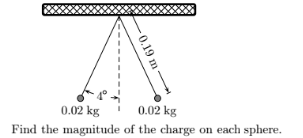# Problem: Two identical small charged spheres hang in equilibrium with equal masses as shown in the figure. The length of the strings are equal and the angle (shown in the figure) with the vertical is identical.The acceleration of gravity is 9.8 m/s 2 and the value of Coulomb’s constant is 8.98755×10 9 N m2/C2.

###### FREE Expert Solution
82% (203 ratings)
###### Problem Details

Two identical small charged spheres hang in equilibrium with equal masses as shown in the figure. The length of the strings are equal and the angle (shown in the figure) with the vertical is identical.

The acceleration of gravity is 9.8 m/s and the value of Coulomb’s constant is 8.98755×10 9 N m2/C2.